# Introduction to double pointers in C - part 1

## Prerequisite knowledge

Before proceeding with this article, you should be comfortable with basic pointers. Use the following resources below to fulfill the prerequisite knowledge:

## Brief review

If we have an integer x declared via

int x = 10;


then x occupies memory at a particular address. This address is just a number, usually represented in hexadecimal. This address can be accessed via &, the "address of" operator.

 1 2 3 4 5 6 7 #include int main(){ int x = 10; printf("The address of x: %p\n", &x); return 0; } 
The address of x: 0x7ffdd657318c


All values in C have a type. The value of &x (the address of the integer x) has the type pointer to int. If x had been declared as a float,&x would have the type pointer to float.

The declaration int *p states "declare p as pointer to int". So p would be a variable that could hold the address of an integer. After being declared, we dereference p using the dereference operator *. (Dereferencing is covered in the C pointer tutorial.)

  1 2 3 4 5 6 7 8 9 10 11 12 #include int main(){ int x = 10; int *p = &x; printf("The address of x: %p\n", &x); printf("The address of x: %p\n", p); printf("The value of x: %d\n", x); printf("The value of x: %d\n", *p); return 0; } 
The address of x: 0x7ffccc5e69e4
The value of x: 10
The value of x: 10


## A basic double pointer

### Storing the address of pointer variables

Suppose we have the declaration

int x = 10;
int *p = &x;


Then p is of type pointer to int, and the value of p is the address of the integer x. Suppose the address of x (denoted &x) is 0x200.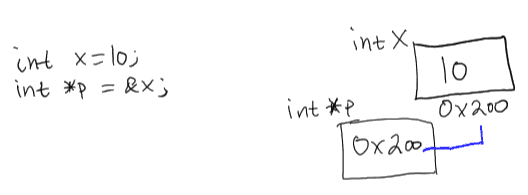The variable p is storing the address of x, which is 0x200. Terminology wise, we would say that "p points to x". Since p is storing a value, and since variables that store values take up space in memory, p must also live at some memory address. Suppose the address of p is 0x450. Since p lives at the address 0x450, we should be able to create a variable - let's call it pp - that stores the address of p.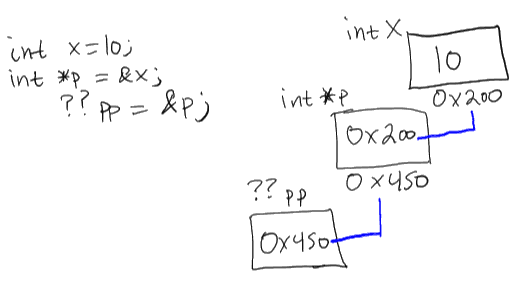So what would the type of &p be? pointer to pointer to int.Let's take a look at a basic source code example.

  1 2 3 4 5 6 7 8 9 10 11 12 13 14 #include int main(){ int x = 10; int *p = &x; int **pp = &p; printf("The address of x: %p\n", &x); printf("The address of x: %p\n", p); printf("-------------------------\n"); printf("The address of p: %p\n", &p); printf("The address of p: %p\n", pp); return 0; } 
The address of x: 0x7ffcc65588ac
-------------------------


A pointer to pointer to int is often called a double pointer to int.

### Dereferencing double pointers

So we've seen that double pointers operate identically to basic pointers in regards to taking the address of a variable. But what about dereferencing a double pointer? Recall that *(&x) == x.

Given the following example:

int x = 10;
int *p = &x;
int **pp = &p;


What would the program output if we were to print out the value of **pp? Let's form some expressions using our fact *(&x) == x.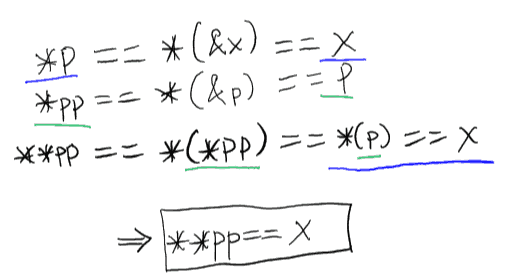Thus the claim implied by working through these expressions is **pp (described as "the double dereference of pp") is equivalent to the variable x for our example above. Here is an alternative visualization of progressing through these expressions.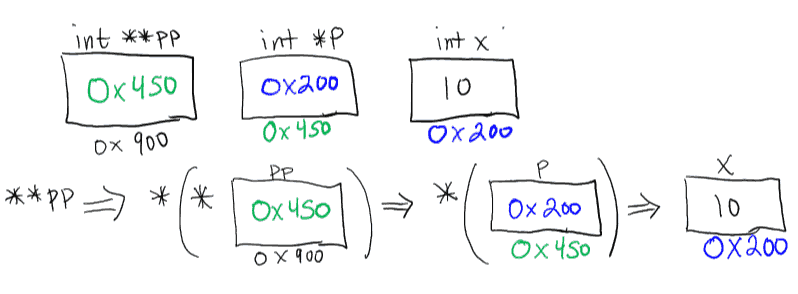Let's verify the claim that **pp == x.

  1 2 3 4 5 6 7 8 9 10 11 12 13 14 15 16 17 18 19 20 21 #include int main(){ int x = 10; int *p = &x; int **pp = &p; if(**pp == x){ printf("We're equivalent!\n\n"); } printf("The value: %d - %d\n", x, **pp); x = 20; printf("The value: %d - %d\n", x, **pp); **pp = 30; printf("The value: %d - %d\n", x, **pp); return 0; } 
We're equivalent!

The value: 10 - 10
The value: 20 - 20
The value: 30 - 30


How did the assignment **pp = 30 change the value of x? Since **pp was equivalent to the variable x, performing the assignment **pp = 30 was equivalent to performing the assignment x = 30.

### Another double dereference example

Let's take a look at a more involved example. Suppose we have the following declarations:

int i = 10;
int j = 25;
int *p1 = &i;
int *p2 = &j;
int **pp = &p2;


We can observe that:

• p1 points to i
• p2 points to j
• pp points to p2

We could visualize the declarations like so:If we were to print out the value of **pp, what would we see? Try to make an educated guess based on the diagram before showing the output.

  1 2 3 4 5 6 7 8 9 10 11 12 13 #include int main(){ int i = 10; int j = 25; int *p1 = &i; int *p2 = &j; int **pp = &p2; printf("The value of **pp: %d\n", **pp); return 0; } 
The value of **pp: 25


Since the value of the pointer pp is the address of p2, we say that "pp points to p2". What happens if we change what pp points to? Will that effect the value of **pp? What if we instructed pp to point to p1 instead of p2?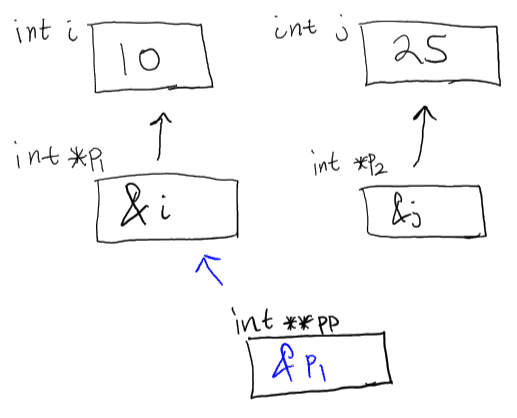1 2 3 4 5 6 7 8 9 10 11 12 13 14 15 16 #include int main(){ int i = 10; int j = 25; int *p1 = &i; int *p2 = &j; int **pp = &p2; // pp now points to p1 pp = &p1; printf("The value of **pp: %d\n", **pp); return 0; } 
The value of **pp: 10


What happens if we change not what pp points to, but the pointer that pp points to? (Which, at this point in time, is p1)1 2 3 4 5 6 7 8 9 10 11 12 13 14 15 16 17 18 19 #include int main(){ int i = 10; int j = 25; int *p1 = &i; int *p2 = &j; int **pp = &p2; // pp now points to p1 pp = &p1; // p1 now points to j p1 = &j; printf("The value of **pp: %d\n", **pp); return 0; } 
The value of **pp: 25


## Type strictness

What type of values can a double pointer to int store? Can they store the address of an int? Or can they only store the address of a pointer to int? Let's experiment!

Suppose we have the following declarations again;

int i = 10;
int j = 25;
int *p1 = &i;
int *p2 = &j;
int **pp = &p2;


Currently, p1 points to the integer i. Is it possible to make p1 point to p2, which is a pointer to int?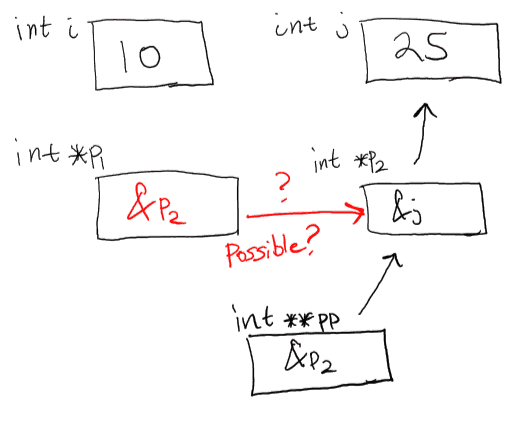Let's try it and see.

  1 2 3 4 5 6 7 8 9 10 11 12 13 14 15 #include int main(){ int i = 10; int j = 25; int *p1 = &i; int *p2 = &j; // Make p1 point to p2 p1 = &p2; printf("The value of *p1: %d\n", *p1); return 0; } 

raw source

Output:

type-strictness-1.c: In function ‘main’:
type-strictness-1.c:10:8: warning: assignment from incompatible pointer type [enabled by default]
p1 = &p2;
^
The value of *p1: 1996267500


Attempting to assign &p2 to p1 results in a warning of assignment from incompatible pointer type. This error means that the pointer variable undergoing assignment is not the correct type to handle the value being assigned. In this case, p2 is not the correct type to handle the address of p1. This is because p2 was declared as a pointer to int, which means it can hold the address of an integer. However, since p1 is a pointer to int, then &p1 is a double pointer to int, so the pointer to int p2 cannot correctly store the double pointer to int &p1. Consequently, dereferencing p1 after the assignment results in some nonsensical value.

NOTE: This is why warning messages can be just as important as error messages in C. Overlooking warning messages can result in disastrous consequences.

What if we were to assign p2 to p1 instead of assigning &p2 to p1? Is that the same as making p1 "point to" p2?

Let's start off with our standard example

int i = 10;
int j = 25;
int *p1 = &i;
int *p2 = &j;


Which we can visualizeNow we reassign the value of p2 to p1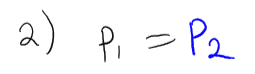What does the assignment look like?This leads us back to our question - Does the assignment p1 = p2 make p1 point to p2? The answer is no. Assignments are only copies of values. In general terms, the assignment x = y means "Store a copy of the value y into the variable x".

Remember, the address of a variable is just some number like 0x7ffdd657318c. If we had a scenario like

int x = 100;
int y = x;
x = 500;


Then we know that the value of y would still be 100. y has no connection to x other than the fact that it received a copy of its value at the time of assignment. Similarly, for the assignment p1 = p2, p1 actually has no connection to p2 whatsoever. All that happened was that p1 received a copy of the value p2 was storing, which happened to be the address of the integer j.

  1 2 3 4 5 6 7 8 9 10 11 12 13 14 15 16 17 18 19 20 #include int main(){ int i = 10; int j = 25; int *p1 = &i; int *p2 = &j; // Reassign p1 to p2, which is the address of j p1 = p2; // Reassign p2 to the address of i p2 = &i; // If p1 actually "points" to p2 then theoretically p1 should now point to i // since we just made p2 point to i. printf("The value of *p1: %d\n", *p1); return 0; } 
The value of *p1: 25


From the output we can conclude that p1 does not point to p2 or have any connection to p2 at all. Assignment between pointer variables of the same type is just like any other type of assignment between variables of the same type - a copy of the value is placed into the variable being assigned to. In the case of pointers, the value just happens to be the memory address of some other variable.

## Tagged as c

Date published - November 14, 2016